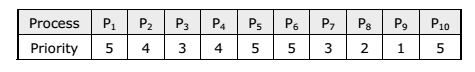# Priority Queue, Heap and Heap Sort A+ A  A-

Heap is a data structure, which permits one to insert elements into a set and also to find the largest element efficiently. A data structure, which provides these two operations, is called a priority queue.

Max and Min Heap data structures:

A max heap is an almost complete binary tree such that the value of each node is greater than or equal to those in its children.Representation of Heap Tree

Since heap is a complete binary tree, a heap tree can be efficiently represented using one dimensional array. This provides a very convenient way of figuring out where children belong to.

• The root of the tree is in location 1.
• The left child of an element stored at location i can be found in location 2*i.
• The right child of an element stored at location i can be found in location 2*i+1.
• The parent of an element stored at location i can be found at location floor(i/2).

The elements of the array can be thought of as lying in a tree structure. A heap tree represented using a single array looks as follows:Operations on heap tree

The major operations required to be performed on a heap tree:

1. Insertion,
2. Deletion and
3. Merging.

Application of heap tree:

They are two main applications of heap trees known are:

1. Sorting (Heap sort) and
2. Priority queue implementation.

HEAP SORT:

A heap sort algorithm works by first organizing the data to be sorted into a special type of binary tree called a heap. Any kind of data can be sorted either in ascending order or in descending order using heap tree. It does this with the following steps:

1. Build a heap tree with the given set of data.

2. Remove the top most item (the largest) and replace it with the last element in the heap.

• Re-heapify the complete binary tree.
• Place the deleted node in the output.

5. Continue step 2 until the heap tree is empty.

Time Complexity:

Each ‘n’ insertion operations takes O(log k), where ‘k’ is the number of elements in the heap at the time. Likewise, each of the ‘n’ remove operations also runs in time O(log k), where ‘k’ is the number of elements in the heap at the time.

Since we always have k ≤ n, each such operation runs in O(log n) time in the worst case.

Thus, for ‘n’ elements it takes O(n log n) time, so the priority queue sorting algorithm runs in O(n log n) time when we use a heap to implement the priority queue.

Priority queue implementation using heap tree:

Priority queue can be implemented using circular array, linked list etc. Another simplified implementation is possible using heap tree; the heap, however, can be represented using an array. This implementation is therefore free from the complexities of circular array and linked list but getting the advantages of simplicities of array. As heap trees allow the duplicity of data in it. Elements associated with their priority values are to be stored in from of heap tree, which can be formed based on their priority values. The top priority element that has to be processed first is at the root; so it can be deleted and heap can be rebuilt to get the next element to be processed, and so on. As an illustration, consider the following processes with their priorities:These processes enter the system in the order as listed above at time 0, say. Assume that a process having higher priority value will be serviced first. The heap tree can be formed considering the process priority values. The order of servicing the process is successive deletion of roots from the heap.